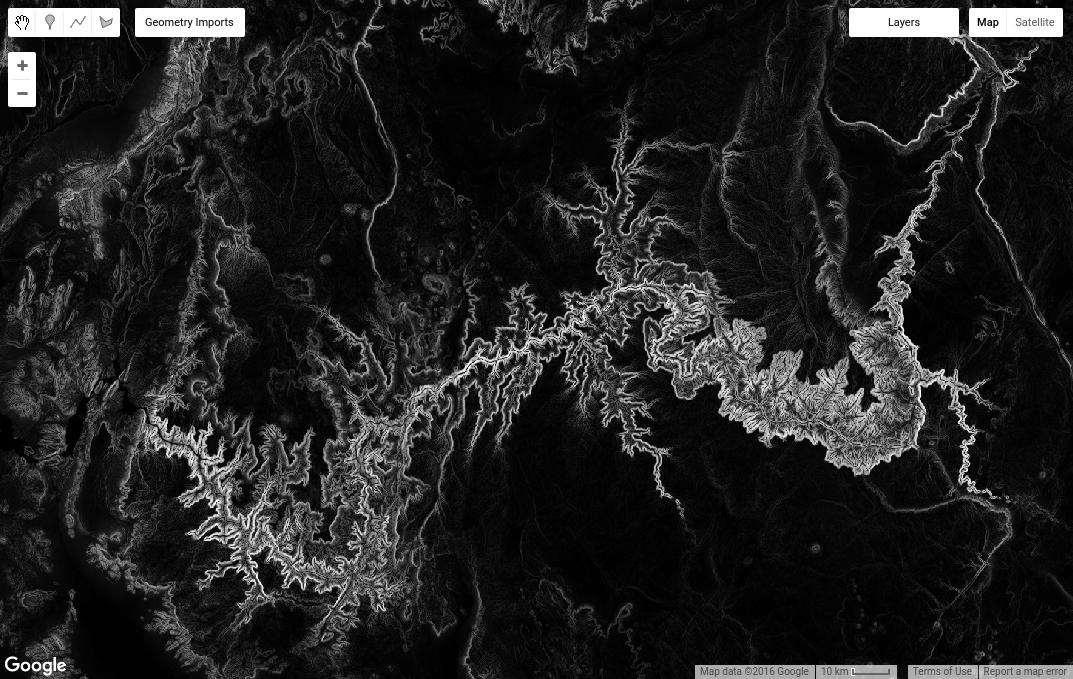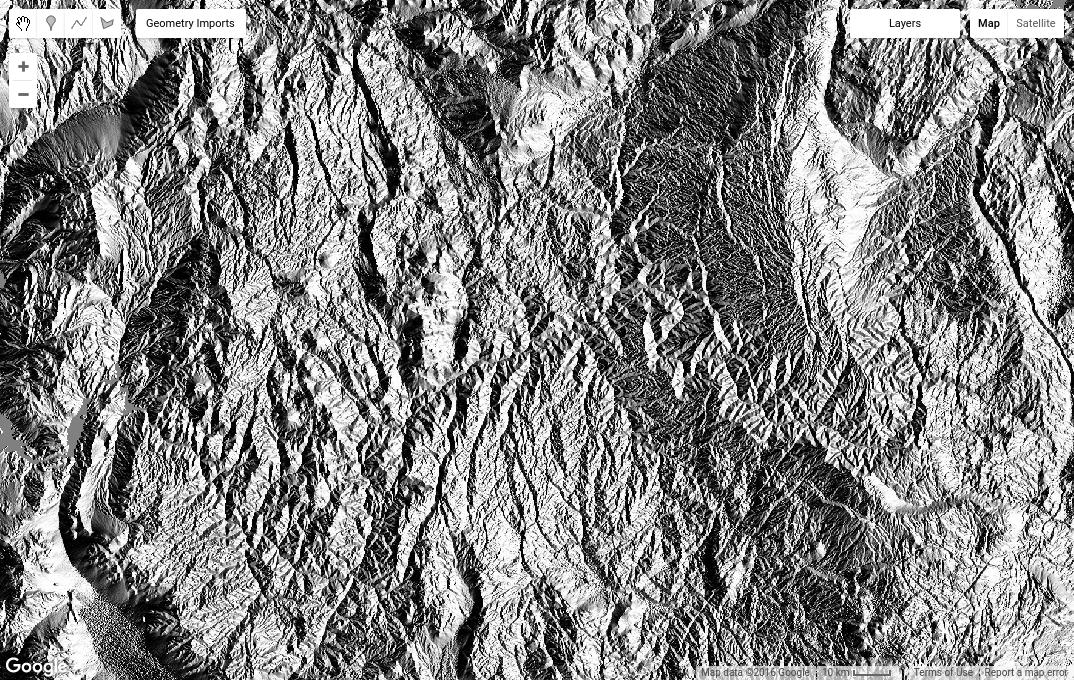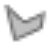# Computations using Images

Now that you know how to load and display an image, it's time to apply a computation to it. For example, you can compute the slope of terrain, by passing the SRTM elevation image to the `slope` method of the `ee.Terrain` package.

```// Load the SRTM image.
var srtm = ee.Image('CGIAR/SRTM90_V4');

// Apply an algorithm to an image.
var slope = ee.Terrain.slope(srtm);

// Display the result.
Map.setCenter(-112.8598, 36.2841, 9); // Center on the Grand Canyon.
Map.addLayer(slope, {min: 0, max :60}, 'slope');
```

Note that in the code `ee.Terrain.slope(srtm)`, the `srtm` image is provided as an argument to the slope algorithm. The result should look something like Figure 3.Figure 3. Slope image.

## Image math

There are also methods in the `ee.Image` class that can be invoked on an image object. For example, suppose you'd like to do some math using image bands (sometimes called band math or map algebra). For example, you may be interested in trigonometric operations on an aspect image. To accomplish that, first convert an aspect image to radians, then call `sin()` on it. Reusing our `srtm` image,

```// Get the aspect (in degrees).
var aspect = ee.Terrain.aspect(srtm);

// Convert to radians, compute the sin of the aspect.
var sinImage = aspect.divide(180).multiply(Math.PI).sin();

// Display the result.
Map.addLayer(sinImage, {min: -1, max: 1}, 'sin');
```

The result should look something like Figure 4. It's worth taking a closer look at the `aspect.divide(180).multiply(Math.PI).sin()` code. By chaining multiple methods like this, the code says, 'divide the aspect by 180, multiply the result of that by π, and finally take the sin'. You can perform complex mathematical operations on images by combining methods in this manner. See the `Image` docs for a complete list of mathematical operations, `add()`, `subtract()`, `multiply()`, etc.Figure 4. Sin of terrain aspect.

## Image statistics

Another useful class of operations on images involves computing pixel statistics in image regions, or raster-vector overlays. To compute statistics in Earth Engine, use a reducer as represented by classes in the `ee.Reducer` package. For example, suppose you're interested in the mean of elevation in some region. You can define a region by drawing a polygon using the geometry drawing tools. To interactively draw a region, get the polygon drawing tool (), then digitize a polygon over your area of interest and click Exit when you're done. Note that the resultant `ee.Geometry` object is automatically named `geometry` and added as an import at the top of your script. Rename that variable to 'polygon' by clicking on the variable name in the imports and typing the new name.

Next, get the mean pixel value in the polygon using the following code:

```// Compute the mean elevation in the polygon.
var meanDict = srtm.reduceRegion({
reducer: ee.Reducer.mean(),
geometry: polygon,
scale: 90
});

// Get the mean from the dictionary and print it.
var mean = meanDict.get('elevation');
print('Mean elevation', mean);
```

There are several things to note here. First, observe that `reduceRegion()` is a method available for `Image` objects (learn more about reducing regions here). Second, the method arguments are provided in a JavaScript object that is passed as a single argument. (Specifically, the keys of the object are the names of the method parameters. The values are the arguments to the method). Third, the `reducer` parameter specifies the type of statistic to compute and the `geometry` parameter specifies the region in which to compute the statistic. The `scale` parameter is the pixel size in meters to use. To avoid ambiguity, you should always specify scale when doing reductions as Earth Engine may not be able to automatically determine the appropriate scale from the inputs. (Learn more about scale in Earth Engine).

Lastly, the return value of `reduceRegion()` is a dictionary in which keys are band names and values are the pixel statistics for the bands. The `get()` method on a dictionary returns the value corresponding to the key provided as an argument. In this case, the `srtm` image has one band, 'elevation', so the example code gets that statistic from the dictionary and prints it.

When you run this code, if you get an error that looks like:

Fear not! There are several things you can do to resolve the error. The `reduceRegion()` method has a check to make sure you consider whether you really want to include so many pixels in your computation. This is intended to prevent you from accidentally doing something silly, like trying compute the mean of every one-meter pixel in the world (don't do that). To resolve the error, either set the `bestEffort` parameter to `true` by adding `bestEffort: true` to the dictionary of parameters, or set the `maxPixels` parameter to a value higher than the default of 10 million pixels, or both. If `bestEffort` is true, Earth Engine will automatically recompute the scale such that `maxPixels` is not exceeded.

## Digression: Scale in Earth Engine

In the previous example, `scale` is set to approximately the native resolution of the SRTM image. You can discover the native resolution of an image with:

```var scale = srtm.projection().nominalScale();
print('SRTM scale in meters', scale);
```

If you specify a scale smaller than the native resolution, Earth Engine will happily resample the input image using nearest neighbor, then include all those smaller pixels in the computation. If you set the scale to be larger, Earth Engine will use input pixels from an aggregated version of the input (i.e. get pixels from a higher level of the image pyramid). Learn more about how Earth Engine handles scale in this doc.

So far, you've been working with a single image with a single band. Now it's time to learn about multi-band images and image collections.